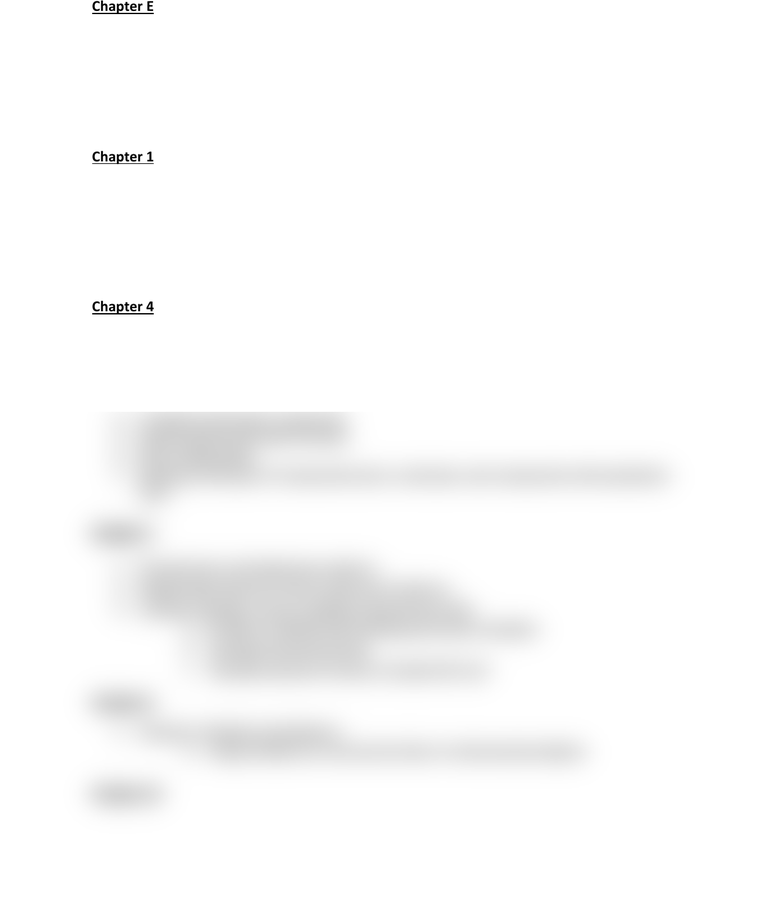Study Guides (390,000)
US (220,000)
UM (1,000)
CHM (50)
Quiz

# CHM 121 Study Guide - Quiz Guide: Molar Mass, Molar Volume, Dimensional Analysis

Department
Chemistry
Course Code
CHM 121
Professor
Cesar Gonzalez
Study Guide
Quiz

This preview shows half of the first page. to view the full 2 pages of the document.Spring 2020 CHM 121 Exam 1 Guide
Chapter E
1. Significant figures and scientific notation
2. Units of Measurements: Conversion factors (metric system)
3. Prefix (you must know the metric system prefix table)
4. Density (units for each phase and how to use in dimensional analysis)
5. Conversion between metric and non-metric, including volume
Chapter 1
1. Classification of matter: states, elements, compounds, mixtures
2. Atomic theory Basics: electrons, protons, neutrons
3. Atomic number % mass number
4. Isotopes/average mass
5. Basics of the mole concept
Chapter 4
1. Basics Periodic table (metals/transition metals/nonmetals)
2. Ions (and polyatomic ions)
3. Ionic compounds
a. Predicting the formula that will form based on two elements combining
4. Covalent (molecular) compounds
5. Empirical and molecular formulas
6. Mole relationships
7. Naming of all types of compounds (ionic, molecular, and compounds with polyatomic
ions)
Chapter 7
1. Stoichiometry and balancing reactions
2. Relationship between moles, grams and reactions
3. Limiting reactants, excess reactants and percent yield
a. Be able to identify both limiting and excess reactants
b. Calculate theoretical yield
c. Calculate amount of excess reactant left over
Chapter 8
1. Solutions: Molarity and dilutions
a. Using molarity as a conversion factor in dimensional analysis
Chapter 10